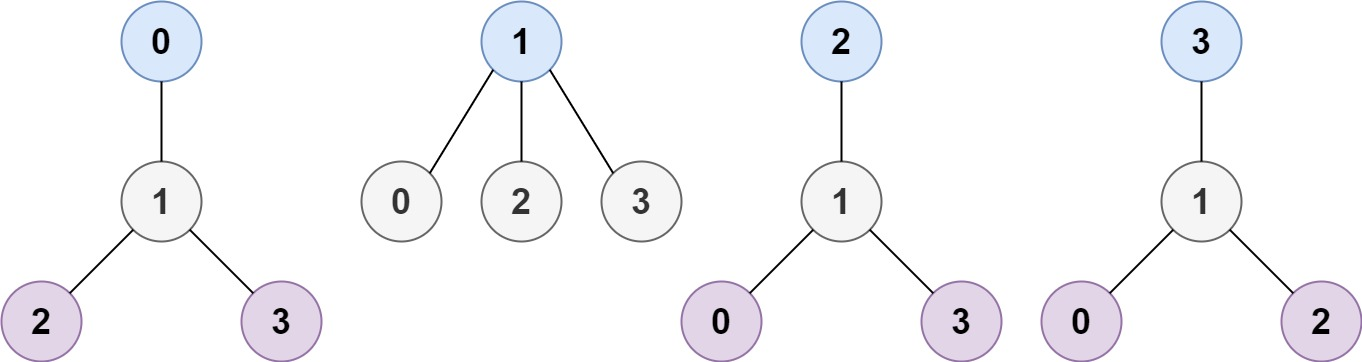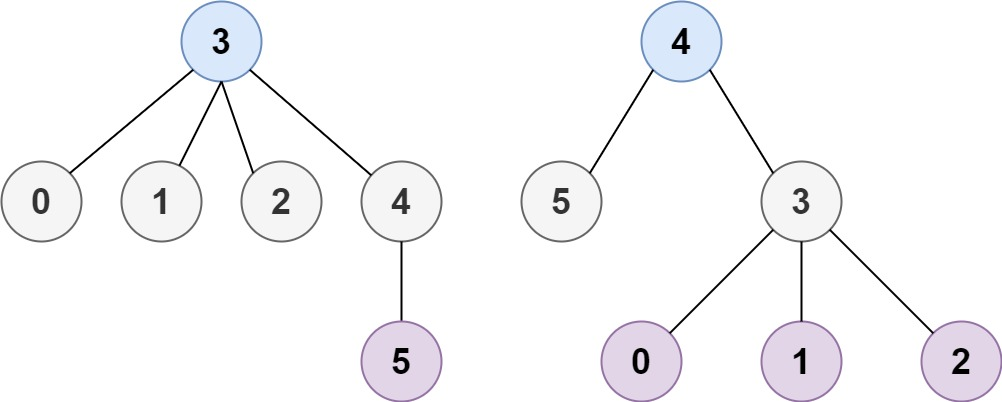# GeetCode Hub

A tree is an undirected graph in which any two vertices are connected by exactly one path. In other words, any connected graph without simple cycles is a tree.

Given a tree of `n` nodes labelled from `0` to `n - 1`, and an array of `n - 1` `edges` where `edges[i] = [ai, bi]` indicates that there is an undirected edge between the two nodes `ai` and `bi` in the tree, you can choose any node of the tree as the root. When you select a node `x` as the root, the result tree has height `h`. Among all possible rooted trees, those with minimum height (i.e. `min(h)`)  are called minimum height trees (MHTs).

Return a list of all MHTs' root labels. You can return the answer in any order.

The height of a rooted tree is the number of edges on the longest downward path between the root and a leaf.

Example 1:```Input: n = 4, edges = [[1,0],[1,2],[1,3]]
Output: 
Explanation: As shown, the height of the tree is 1 when the root is the node with label 1 which is the only MHT.
```

Example 2:```Input: n = 6, edges = [[3,0],[3,1],[3,2],[3,4],[5,4]]
Output: [3,4]
```

Example 3:

```Input: n = 1, edges = []
Output: 
```

Example 4:

```Input: n = 2, edges = [[0,1]]
Output: [0,1]
```

Constraints:

• `1 <= n <= 2 * 104`
• `edges.length == n - 1`
• `0 <= ai, bi < n`
• `ai != bi`
• All the pairs `(ai, bi)` are distinct.
• The given input is guaranteed to be a tree and there will be no repeated edges.

class Solution { public List<Integer> findMinHeightTrees(int n, int[][] edges) { } }